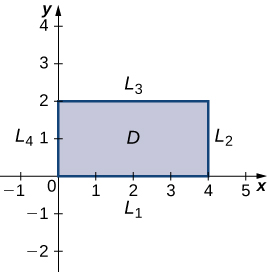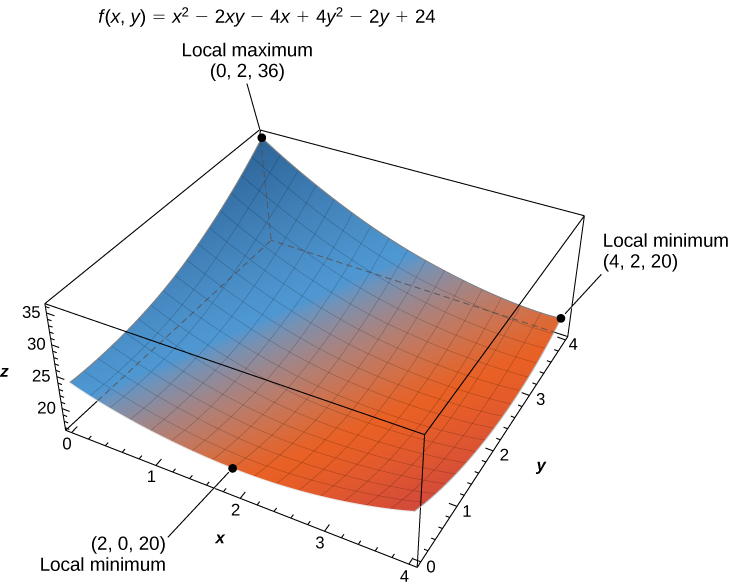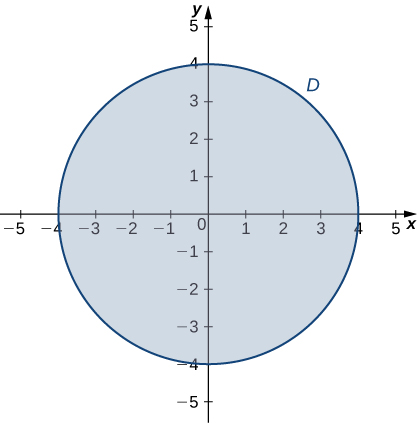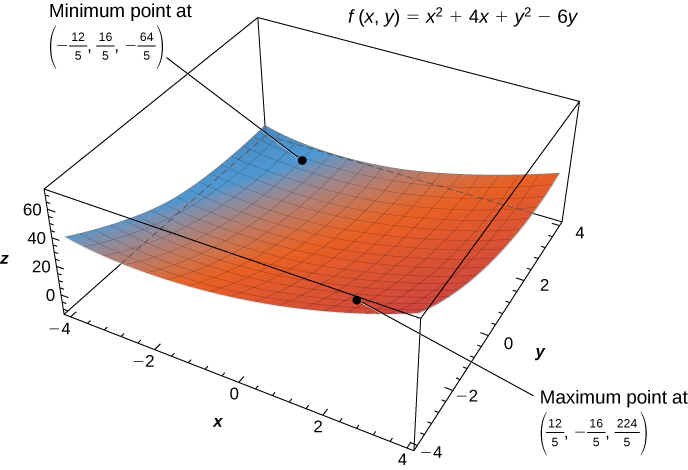# 4.7 Maxima/minima problems  (Page 5/10)

 Page 5 / 10

If the boundary of the set $D$ is a more complicated curve defined by a function $g\left(x,y\right)=c$ for some constant $c,$ and the first-order partial derivatives of $g$ exist, then the method of Lagrange multipliers can prove useful for determining the extrema of $f$ on the boundary. The method of Lagrange multipliers is introduced in Lagrange Multipliers .

## Finding absolute extrema

Use the problem-solving strategy for finding absolute extrema of a function to determine the absolute extrema of each of the following functions:

1. $f\left(x,y\right)={x}^{2}-2xy+4{y}^{2}-4x-2y+24$ on the domain defined by $0\le x\le 4$ and $0\le y\le 2$
2. $g\left(x,y\right)={x}^{2}+{y}^{2}+4x-6y$ on the domain defined by ${x}^{2}+{y}^{2}\le 16$
1. Using the problem-solving strategy, step $1$ involves finding the critical points of $f$ on its domain. Therefore, we first calculate ${f}_{x}\left(x,y\right)$ and ${f}_{y}\left(x,y\right),$ then set them each equal to zero:
$\begin{array}{ccc}\hfill {f}_{x}\left(x,y\right)& =\hfill & 2x-2y-4\hfill \\ \hfill {f}_{y}\left(x,y\right)& =\hfill & -2x+8y-2.\hfill \end{array}$

Setting them equal to zero yields the system of equations
$\begin{array}{ccc}\hfill 2x-2y-4& =\hfill & 0\hfill \\ \hfill -2x+8y-2& =\hfill & 0.\hfill \end{array}$

The solution to this system is $x=3$ and $y=1.$ Therefore $\left(3,1\right)$ is a critical point of $f.$ Calculating $f\left(3,1\right)$ gives $f\left(3,1\right)=17.$
The next step involves finding the extrema of $f$ on the boundary of its domain. The boundary of its domain consists of four line segments as shown in the following graph:Graph of the domain of the function f ( x , y ) = x 2 − 2 x y + 4 y 2 − 4 x − 2 y + 24 .
${L}_{1}$ is the line segment connecting $\left(0,0\right)$ and $\left(4,0\right),$ and it can be parameterized by the equations $x\left(t\right)=t,y\left(t\right)=0$ for $0\le t\le 4.$ Define $g\left(t\right)=f\left(x\left(t\right),y\left(t\right)\right).$ This gives $g\left(t\right)={t}^{2}-4t+24.$ Differentiating g leads to ${g}^{\prime }\left(t\right)=2t-4.$ Therefore, $g$ has a critical value at $t=2,$ which corresponds to the point $\left(2,0\right).$ Calculating $f\left(2,0\right)$ gives the z- value $20.$
${L}_{2}$ is the line segment connecting $\left(4,0\right)$ and $\left(4,2\right),$ and it can be parameterized by the equations $x\left(t\right)=4,y\left(t\right)=t$ for $0\le t\le 2.$ Again, define $g\left(t\right)=f\left(x\left(t\right),y\left(t\right)\right).$ This gives $g\left(t\right)=4{t}^{2}-10t+24.$ Then, ${g}^{\prime }\left(t\right)=8t-10.$ $g$ has a critical value at $t=\frac{5}{4},$ which corresponds to the point $\left(0,\frac{5}{4}\right).$ Calculating $f\left(0,\frac{5}{4}\right)$ gives the z- value $27.75.$
${L}_{3}$ is the line segment connecting $\left(0,2\right)$ and $\left(4,2\right),$ and it can be parameterized by the equations $x\left(t\right)=t,y\left(t\right)=2$ for $0\le t\le 4.$ Again, define $g\left(t\right)=f\left(x\left(t\right),y\left(t\right)\right).$ This gives $g\left(t\right)={t}^{2}-8t+36.$ The critical value  corresponds to the point $\left(4,2\right).$ So, calculating $f\left(4,2\right)$ gives the z- value $20.$
${L}_{4}$ is the line segment connecting $\left(0,0\right)$ and $\left(0,2\right),$ and it can be parameterized by the equations $x\left(t\right)=0,y\left(t\right)=t$ for $0\le t\le 2.$ This time, $g\left(t\right)=4{t}^{2}-2t+24$ and the critical value $t=\frac{1}{4}$ correspond to the point $\left(0,\frac{1}{4}\right).$ Calculating $f\left(0,\frac{1}{4}\right)$ gives the z- value $23.75.$
We also need to find the values of $f\left(x,y\right)$ at the corners of its domain. These corners are located at $\left(0,0\right),\left(4,0\right),\left(4,2\right)\phantom{\rule{0.2em}{0ex}}\text{and}\phantom{\rule{0.2em}{0ex}}\left(0,2\right)\text{:}$
$\begin{array}{}\\ \hfill f\left(0,0\right)& =\hfill & {\left(0\right)}^{2}-2\left(0\right)\left(0\right)+4{\left(0\right)}^{2}-4\left(0\right)-2\left(0\right)+24\hfill & =\hfill & 24\hfill \\ \hfill f\left(4,0\right)& =\hfill & {\left(4\right)}^{2}-2\left(4\right)\left(0\right)+4{\left(0\right)}^{2}-4\left(4\right)-2\left(0\right)+24\hfill & =\hfill & 24\hfill \\ \hfill f\left(4,2\right)& =\hfill & {\left(4\right)}^{2}-2\left(4\right)\left(2\right)+4{\left(2\right)}^{2}-4\left(4\right)-2\left(2\right)+24\hfill & =\hfill & 20\hfill \\ \hfill f\left(0,2\right)& =\hfill & {\left(0\right)}^{2}-2\left(0\right)\left(2\right)+4{\left(2\right)}^{2}-4\left(0\right)-2\left(2\right)+24\hfill & =\hfill & 36.\hfill \end{array}$

The absolute maximum value is $36,$ which occurs at $\left(0,2\right),$ and the global minimum value is $20,$ which occurs at both $\left(4,2\right)$ and $\left(2,0\right)$ as shown in the following figure.The function f ( x , y ) has two global minima and one global maximum over its domain.
2. Using the problem-solving strategy, step $1$ involves finding the critical points of $g$ on its domain. Therefore, we first calculate ${g}_{x}\left(x,y\right)$ and ${g}_{y}\left(x,y\right),$ then set them each equal to zero:
$\begin{array}{ccc}\hfill {g}_{x}\left(x,y\right)& =\hfill & 2x+4\hfill \\ \hfill {g}_{y}\left(x,y\right)& =\hfill & 2y-6.\hfill \end{array}$

Setting them equal to zero yields the system of equations
$\begin{array}{ccc}\hfill 2x+4& =\hfill & 0\hfill \\ \hfill 2y-6& =\hfill & 0.\hfill \end{array}$

The solution to this system is $x=-2$ and $y=3.$ Therefore, $\left(-2,3\right)$ is a critical point of $g.$ Calculating $g\left(-2,3\right),$ we get
$g\left(-2,3\right)={\left(-2\right)}^{2}+{3}^{2}+4\left(-2\right)-6\left(3\right)=4+9-8-18=-13.$

The next step involves finding the extrema of g on the boundary of its domain. The boundary of its domain consists of a circle of radius $4$ centered at the origin as shown in the following graph.Graph of the domain of the function g ( x , y ) = x 2 + y 2 + 4 x − 6 y .
The boundary of the domain of $g$ can be parameterized using the functions $x\left(t\right)=4\phantom{\rule{0.2em}{0ex}}\text{cos}\phantom{\rule{0.2em}{0ex}}t,y\left(t\right)=4\phantom{\rule{0.2em}{0ex}}\text{sin}\phantom{\rule{0.2em}{0ex}}t$ for $0\le t\le 2\pi .$ Define $h\left(t\right)=g\left(x\left(t\right),y\left(t\right)\right)\text{:}$
$\begin{array}{cc}\hfill h\left(t\right)& =g\left(x\left(t\right),y\left(t\right)\right)\hfill \\ & ={\left(4\phantom{\rule{0.2em}{0ex}}\text{cos}\phantom{\rule{0.2em}{0ex}}t\right)}^{2}+{\left(4\phantom{\rule{0.2em}{0ex}}\text{sin}\phantom{\rule{0.2em}{0ex}}t\right)}^{2}+4\left(4\phantom{\rule{0.2em}{0ex}}\text{cos}\phantom{\rule{0.2em}{0ex}}t\right)-6\left(4\phantom{\rule{0.2em}{0ex}}\text{sin}\phantom{\rule{0.2em}{0ex}}t\right)\hfill \\ & =16{\text{cos}}^{2}\phantom{\rule{0.1em}{0ex}}t+16{\text{sin}}^{2}\phantom{\rule{0.1em}{0ex}}t+16\phantom{\rule{0.2em}{0ex}}\text{cos}\phantom{\rule{0.2em}{0ex}}t-24\phantom{\rule{0.2em}{0ex}}\text{sin}\phantom{\rule{0.2em}{0ex}}t\hfill \\ & =16+16\phantom{\rule{0.2em}{0ex}}\text{cos}\phantom{\rule{0.2em}{0ex}}t-24\phantom{\rule{0.2em}{0ex}}\text{sin}\phantom{\rule{0.2em}{0ex}}t.\hfill \end{array}$

Setting ${h}^{\prime }\left(t\right)=0$ leads to
$\begin{array}{ccc}\hfill -16\phantom{\rule{0.2em}{0ex}}\text{sin}\phantom{\rule{0.2em}{0ex}}t-24\phantom{\rule{0.2em}{0ex}}\text{cos}\phantom{\rule{0.2em}{0ex}}t& =\hfill & 0\hfill \\ \hfill -16\phantom{\rule{0.2em}{0ex}}\text{sin}\phantom{\rule{0.2em}{0ex}}t& =\hfill & 24\phantom{\rule{0.2em}{0ex}}\text{cos}\phantom{\rule{0.2em}{0ex}}t\hfill \\ \hfill \frac{-16\phantom{\rule{0.2em}{0ex}}\text{sin}\phantom{\rule{0.2em}{0ex}}t}{-16\phantom{\rule{0.2em}{0ex}}\text{cos}\phantom{\rule{0.2em}{0ex}}t}& =\hfill & \frac{24\phantom{\rule{0.2em}{0ex}}\text{cos}\phantom{\rule{0.2em}{0ex}}t}{-16\phantom{\rule{0.2em}{0ex}}\text{cos}\phantom{\rule{0.2em}{0ex}}t}\hfill \\ \hfill \text{tan}\phantom{\rule{0.2em}{0ex}}t& =\hfill & -\frac{4}{3}.\hfill \end{array}$

$\begin{array}{ccc}\hfill -16\phantom{\rule{0.2em}{0ex}}\text{sin}\phantom{\rule{0.2em}{0ex}}t-24\phantom{\rule{0.2em}{0ex}}\text{cos}\phantom{\rule{0.2em}{0ex}}t& =\hfill & 0\hfill \\ \hfill -16\phantom{\rule{0.2em}{0ex}}\text{sin}\phantom{\rule{0.2em}{0ex}}t& =\hfill & 24\phantom{\rule{0.2em}{0ex}}\text{cos}\phantom{\rule{0.2em}{0ex}}t\hfill \\ \hfill \frac{-16\phantom{\rule{0.2em}{0ex}}\text{sin}\phantom{\rule{0.2em}{0ex}}t}{-16\phantom{\rule{0.2em}{0ex}}\text{cos}\phantom{\rule{0.2em}{0ex}}t}& =\hfill & \frac{24\phantom{\rule{0.2em}{0ex}}\text{cos}\phantom{\rule{0.2em}{0ex}}t}{-16\phantom{\rule{0.2em}{0ex}}\text{cos}\phantom{\rule{0.2em}{0ex}}t}\hfill \\ \hfill \text{tan}\phantom{\rule{0.2em}{0ex}}t& =\hfill & -\frac{3}{2}.\hfill \end{array}$

This equation has two solutions over the interval $0\le t\le 2\pi .$ One is $t=\pi -\text{arctan}\left(\frac{3}{2}\right)$ and the other is $t=2\pi -\text{arctan}\left(\frac{3}{2}\right).$ For the first angle,
$\begin{array}{ccc}\hfill \text{sin}\phantom{\rule{0.2em}{0ex}}t& =\hfill & \text{sin}\left(\pi -\text{arctan}\left(\frac{3}{2}\right)\right)=\text{sin}\left(\text{arctan}\left(\frac{3}{2}\right)\right)=\frac{3\sqrt{13}}{13}\hfill \\ \hfill \text{cos}\phantom{\rule{0.2em}{0ex}}t& =\hfill & \text{cos}\left(\pi -\text{arctan}\left(\frac{3}{2}\right)\right)=\text{−}\text{cos}\left(\text{arctan}\left(\frac{3}{2}\right)\right)=-\frac{2\sqrt{13}}{13}.\hfill \end{array}$

Therefore, $x\left(t\right)=4\phantom{\rule{0.2em}{0ex}}\text{cos}\phantom{\rule{0.2em}{0ex}}t=-\frac{8\sqrt{13}}{13}$ and $y\left(t\right)=4\phantom{\rule{0.2em}{0ex}}\text{sin}\phantom{\rule{0.2em}{0ex}}t=\frac{12\sqrt{13}}{13},$ so $\left(-\frac{8\sqrt{13}}{13},\frac{12\sqrt{13}}{13}\right)$ is a critical point on the boundary and
$\begin{array}{cc}\hfill g\left(-\frac{8\sqrt{13}}{13},\frac{12\sqrt{13}}{13}\right)& ={\left(-\frac{8\sqrt{13}}{13}\right)}^{2}+{\left(\frac{12\sqrt{13}}{13}\right)}^{2}+4\left(-\frac{8\sqrt{13}}{13}\right)-6\left(\frac{12\sqrt{13}}{13}\right)\hfill \\ & =\frac{144}{13}+\frac{64}{13}-\frac{32\sqrt{13}}{13}-\frac{72\sqrt{13}}{13}\hfill \\ & =\frac{208-104\sqrt{13}}{13}\approx -12.844.\hfill \end{array}$

For the second angle,
$\begin{array}{ccc}\hfill \text{sin}\phantom{\rule{0.2em}{0ex}}t& =\hfill & \text{sin}\left(2\pi -\text{arctan}\left(\frac{3}{2}\right)\right)=\text{−}\text{sin}\left(\text{arctan}\left(\frac{3}{2}\right)\right)=-\frac{3\sqrt{13}}{13}\hfill \\ \hfill \text{cos}\phantom{\rule{0.2em}{0ex}}t& =\hfill & \text{cos}\left(2\pi -\text{arctan}\left(\frac{3}{2}\right)\right)=\text{cos}\left(\text{arctan}\left(\frac{3}{2}\right)\right)=\frac{2\sqrt{13}}{13}.\hfill \end{array}$

Therefore, $x\left(t\right)=4\phantom{\rule{0.2em}{0ex}}\text{cos}\phantom{\rule{0.2em}{0ex}}t=\frac{8\sqrt{13}}{13}$ and $y\left(t\right)=4\phantom{\rule{0.2em}{0ex}}\text{sin}\phantom{\rule{0.2em}{0ex}}t=-\frac{12\sqrt{13}}{13},$ so $\left(\frac{8\sqrt{13}}{13},-\frac{12\sqrt{13}}{13}\right)$ is a critical point on the boundary and
$\begin{array}{cc}\hfill g\left(\frac{8\sqrt{13}}{13},-\frac{12\sqrt{13}}{13}\right)& ={\left(\frac{8\sqrt{13}}{13}\right)}^{2}+{\left(-\frac{12\sqrt{13}}{13}\right)}^{2}+4\left(\frac{8\sqrt{13}}{13}\right)-6\left(-\frac{12\sqrt{13}}{13}\right)\hfill \\ & =\frac{144}{13}+\frac{64}{13}+\frac{32\sqrt{13}}{13}+\frac{72\sqrt{13}}{13}\hfill \\ & =\frac{208+104\sqrt{13}}{13}\approx 44.844.\hfill \end{array}$

The absolute minimum of g is $-13,$ which is attained at the point $\left(-2,3\right),$ which is an interior point of D . The absolute maximum of g is approximately equal to 44.844, which is attained at the boundary point $\left(\frac{8\sqrt{13}}{13},-\frac{12\sqrt{13}}{13}\right).$ These are the absolute extrema of g on D as shown in the following figure.The function f ( x , y ) has a local minimum and a local maximum.

#### Questions & Answers

how can chip be made from sand
Eke Reply
is this allso about nanoscale material
Almas
are nano particles real
Missy Reply
yeah
Joseph
Hello, if I study Physics teacher in bachelor, can I study Nanotechnology in master?
Lale Reply
no can't
Lohitha
where is the latest information on a no technology how can I find it
William
currently
William
where we get a research paper on Nano chemistry....?
Maira Reply
nanopartical of organic/inorganic / physical chemistry , pdf / thesis / review
Ali
what are the products of Nano chemistry?
Maira Reply
There are lots of products of nano chemistry... Like nano coatings.....carbon fiber.. And lots of others..
learn
Even nanotechnology is pretty much all about chemistry... Its the chemistry on quantum or atomic level
learn
Google
da
no nanotechnology is also a part of physics and maths it requires angle formulas and some pressure regarding concepts
Bhagvanji
hey
Giriraj
Preparation and Applications of Nanomaterial for Drug Delivery
Hafiz Reply
revolt
da
Application of nanotechnology in medicine
has a lot of application modern world
Kamaluddeen
yes
narayan
what is variations in raman spectra for nanomaterials
Jyoti Reply
ya I also want to know the raman spectra
Bhagvanji
I only see partial conversation and what's the question here!
Crow Reply
what about nanotechnology for water purification
RAW Reply
please someone correct me if I'm wrong but I think one can use nanoparticles, specially silver nanoparticles for water treatment.
Damian
yes that's correct
Professor
I think
Professor
Nasa has use it in the 60's, copper as water purification in the moon travel.
Alexandre
nanocopper obvius
Alexandre
what is the stm
Brian Reply
is there industrial application of fullrenes. What is the method to prepare fullrene on large scale.?
Rafiq
industrial application...? mmm I think on the medical side as drug carrier, but you should go deeper on your research, I may be wrong
Damian
How we are making nano material?
LITNING Reply
what is a peer
LITNING Reply
What is meant by 'nano scale'?
LITNING Reply
What is STMs full form?
LITNING
scanning tunneling microscope
Sahil
how nano science is used for hydrophobicity
Santosh
Do u think that Graphene and Fullrene fiber can be used to make Air Plane body structure the lightest and strongest. Rafiq
Rafiq
what is differents between GO and RGO?
Mahi
what is simplest way to understand the applications of nano robots used to detect the cancer affected cell of human body.? How this robot is carried to required site of body cell.? what will be the carrier material and how can be detected that correct delivery of drug is done Rafiq
Rafiq
if virus is killing to make ARTIFICIAL DNA OF GRAPHENE FOR KILLED THE VIRUS .THIS IS OUR ASSUMPTION
Anam
analytical skills graphene is prepared to kill any type viruses .
Anam
Any one who tell me about Preparation and application of Nanomaterial for drug Delivery
Hafiz
what is Nano technology ?
Bob Reply
write examples of Nano molecule?
Bob
The nanotechnology is as new science, to scale nanometric
brayan
nanotechnology is the study, desing, synthesis, manipulation and application of materials and functional systems through control of matter at nanoscale
Damian
can you provide the details of the parametric equations for the lines that defince doubly-ruled surfeces (huperbolids of one sheet and hyperbolic paraboloid). Can you explain each of the variables in the equations?
Radek Reply

### Read also:

#### Get Jobilize Job Search Mobile App in your pocket Now!

Source:  OpenStax, Calculus volume 3. OpenStax CNX. Feb 05, 2016 Download for free at http://legacy.cnx.org/content/col11966/1.2
Google Play and the Google Play logo are trademarks of Google Inc.

Notification Switch

Would you like to follow the 'Calculus volume 3' conversation and receive update notifications?By Yacoub JayoghliBy Madison ChristianBy Brooke DelaneyBy Nicole BartelsBy Brooke DelaneyBy OpenStaxByBy Madison ChristianBy Lakeima RobertsBy OpenStax